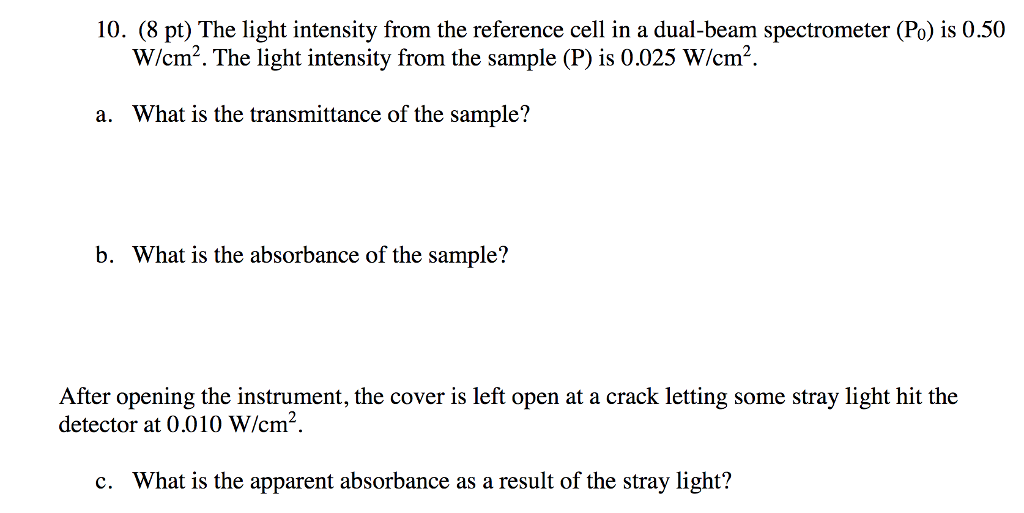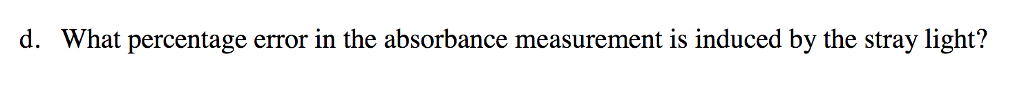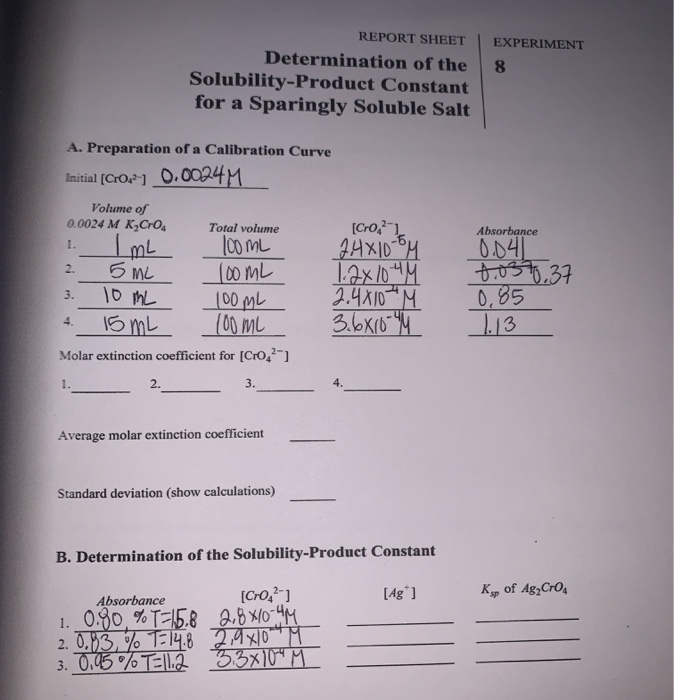# 10. (8 pt) The light intensity from the reference cell in a dual-beam spectrometer (Po) is...10. (8 pt) The light intensity from the reference cell in a dual-beam spectrometer (Po) is 0.50 W/cm2. The light intensity from the sample (P) is 0.025 W/cm. a. What is the transmittance of the sample? b. What is the absorbance of the sample? After opening the instrument, the cover is left open at a crack letting some stray light hit the detector at 0.010 W/cm2 c. What is the apparent absorbance as a result of the stray light?

10. a) Tranamittance (T) = I/Io =

I = intensity of the light after passing through sample. I0 = intensity of the incident light.

T = 0.025/0.5 = 0.05

B) absorbance A = - log T = - log 0.05 = 1.30

C) when stray light is used

Incident intensity and intensity after absorption both changes

SL is intensity of stray light.

Absorbance (A) = -log (I + SL)/(I0+ SL)

= -log ( 0.025+0.010/0.5+0.010)

= - log( 0.035/0.51) = 1.163

D)

Perecentage error = |(measured - accepted)| *100/ accepted

( 1.30-1.163)*100/1.30 = 10.53 %

##### Add Answer to: 10. (8 pt) The light intensity from the reference cell in a dual-beam spectrometer (Po) is...help please? this was the only other information given REPORT SHEET Determination of the Solubility-Product Constant for a Sparingly Soluble Salt EXPERIMENT 8 A. Preparation of a Calibration Curve Initial (Cro121 0.0024 M Absorbance 5 mL Volume of 0.0024 M K Cro Total volume 1. I mL 100 mL 2. 100ML 3. 10 mL 100ml 4. 15 mL 100 ML Molar extinction coefficient for [CrO2) [Cro,2) 2.4x100M 12x1044 2.4810M 3.6810M 0.04) 2037.37 0.85 1.13 2. 3. Average molar extinction coefficient...